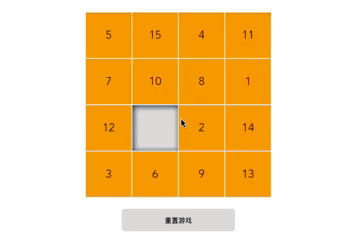# 效果展示# 关键代码

## 游戏面板的构建

<!-- HTML 部分 -->
<ul class="puzzle-wrap">
<li
:class="{'puzzle': true, 'puzzle-empty': !puzzle}"
v-for="(puzzle, index) in puzzles"
:key="index"
v-text="puzzle"
></li>
</ul>

// 数据部分
export default {
data() {
return {
puzzles: Array.from({ length: 15 }, (value, index) => index + 1)
}
},
}


function shuffle(arr) {
let len = arr.length

for (let i = 0; i < len - 1; i++) {
let idx = Math.floor(Math.random() * (len - i))
let temp = arr[idx]
arr[idx] = arr[len - i - 1]
arr[len - i - 1] = temp
}

return arr
}


• 第一步算出 m-n 的值，假设等于 w

• 第二步 Math.random() * w

• 第三步 Math.random() * w + n

• 第四步 Math.floor(Math.random() * w + n)

this.puzzles.push('');


# 交换方块位置

export default {
methods: {

// 点击方块
moveFn(index) {
let puzzles = this.puzzles

// 获取点击位置上下左右的值
let leftNum = this.puzzles[index - 1],
rightNum = this.puzzles[index + 1],
topNum = this.puzzles[index - 4],
bottomNum = this.puzzles[index + 4]

// 和为空的位置交换数值
if (leftNum === '' && index % 4) {
this.setPuzzle(index, -1)
} else if (rightNum === '' && 3 !== index % 4) {
this.setPuzzle(index, 1)
} else if (topNum === '') {
this.setPuzzle(index, -4)
} else if (bottomNum === '') {
this.setPuzzle(index, 4)
}
},

// 设置数组值
setPuzzle(index, num) {
let curNum = this.puzzles[index]

this.$set(this.puzzles, index + num, curNum) this.$set(this.puzzles, index, '')
},
}
}


## 为什么要用 $set 方法 大家应该都知道如果不用$set 方法我们可以直接通过操作数组索引的形式对数组进行赋值，从而交换拼图的中两者的数据：

// 设置数组值
setPuzzle(index, num) {
let curNum = this.puzzles[index]

this.puzzles[index + num] = curNum
this.puzzles[index] = ''

// this.$set(this.puzzles, index + num, curNum) // this.$set(this.puzzles, index, '')
}


• 当你利用索引直接设置一个项时，例如：vm.items[indexOfItem] = newValue

• 当你修改数组的长度时，例如：vm.items.length = newLength

// 设置数组值
setPuzzle(index, num) {
let curNum = this.puzzles[index]

this.puzzles[index + num] = curNum
this.puzzles[index] = ''

this.$forceUpdate() // 迫使 Vue 实例重新渲染 // this.$set(this.puzzles, index + num, curNum)
// this.$set(this.puzzles, index, '') }  上方我们使用了 Vue 提供的$forceUpdate 方法迫使 Vue 实例重新渲染，这样改变的数据就会被更新的页面中去。

## 为什么不用 sort 排序

let puzzleArr = Array.from({ length: 15 }, (value, index) => index + 1);

// 随机打乱数组
puzzleArr = puzzleArr.sort(() => {
return Math.random() - 0.5
});


# 思考 & 作业

Vue 中监听数据变化的原理是什么？是通过何种方式实现的？

# 参考资料

https://www.kancloud.cn/sllyli/vueproject/1244263